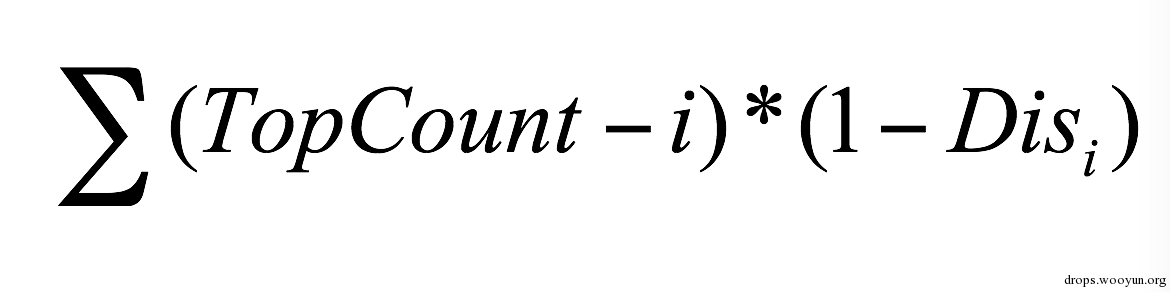# 0x02 验证码识别原理与过程

## 预处理

### 常用的方法：阈值法``````#!python
def Binarized(Picture):
(Width, Height) = Picture.size

Threshold = 80    # 阈值

for i in xrange(Width):
for j in xrange(Height):
if Pixels[i, j] > Threshold: # 大于阈值的置为背景色，否则置为前景色（文字的颜色）
Pixels[i, j] = BACKCOLOR
else:
Pixels[i, j] = TEXTCOLOR
return Picture
``````

### 去除干扰``````#!python
def Enhance(Picture):
'''分离有效信息和干扰信息'''
Result = Picture.copy()
(Width, Height) = Picture.size

xx = [1, 0, -1, 0, 1, -1, 1, -1]
yy = [0, 1, 0, -1, -1, 1, 1, -1]

Threshold = 50

Window = []
for i in xrange(Width):
for j in xrange(Height):
Window = [i, j]
for k in xrange(8):  # 取3*3窗口中像素值存在Window中
if 0 <= i + xx[k] < Width and 0 <= j + yy[k] < Height:
Window.append((i + xx[k], j + yy[k]))
Window.sort()
(x, y) = Window[len(Window) / 2]
if (abs(Pixels[x, y] - Pixels[i, j]) < Threshold):    # 若差值小于阈值则进行“强化”
if Pixels[i, j] < 255 - Pixels[i,j]:   # 若该点接近黑色则将其置为黑色（0），否则置为白色（255）
ResultPixels[i, j] = 0
else:
ResultPixels[i, j] = 255
else:
ResultPixels[i, j] = Pixels[i, j]
return Result
``````

### 降噪``````#!python
def Smooth(Picture):
'''平滑降噪'''
(Width, Height) = Picture.size

xx = [1, 0, -1, 0]
yy = [0, 1, 0, -1]

for i in xrange(Width):
for j in xrange(Height):
if Pixels[i, j] != BACKCOLOR:
Count = 0
for k in xrange(4):
try:
if Pixels[i + xx[k], j + yy[k]] == BACKCOLOR:
Count += 1
except IndexError: # 忽略访问越界的情况
pass
if Count > 3:
Pixels[i, j] = BACKCOLOR
return Picture
``````

## 字符分割

### 投影法### 连通区域法### 对粘连字符的处理

#### 1. 根据平均字符宽度找极小值点分割字符#### 2. 滴水算法``````#!python
def SplitCharacter(Block):
'''根据平均字符宽度找极小值点分割字符'''
(Width, Height) = Block.size
MaxWidth = 20 # 最大字符宽度
MeanWidth = 14    # 平均字符宽度
if Width < MaxWidth:  # 若小于最大字符宽度则认为是单个字符
return [Block]
Blocks = []
PixelCount = []
for i in xrange(Width):  # 统计竖直方向像素个数
Count = 0
for j in xrange(Height):
if Pixels[i, j] == TEXTCOLOR:
Count += 1
PixelCount.append(Count)

for i in xrange(Width):  # 从平均字符宽度处向两侧找极小值点，从极小值点处进行分割
if MeanWidth - i > 0:
if PixelCount[MeanWidth - i - 1] > PixelCount[MeanWidth - i] < PixelCount[MeanWidth - i + 1]:
Blocks.append(Block.crop((0, 0, MeanWidth - i + 1, Height)))
Blocks += SplitCharacter(Block.crop((MeanWidth - i + 1, 0, Width, Height)))
break
if MeanWidth + i < Width - 1:
if PixelCount[MeanWidth + i - 1] > PixelCount[MeanWidth + i] < PixelCount[MeanWidth + i + 1]:
Blocks.append(Block.crop((0, 0, MeanWidth + i + 1, Height)))
Blocks += SplitCharacter(Block.crop((MeanWidth + i + 1, 0, Width, Height)))
break
return Blocks

#!python
def SplitPicture(Picture):
'''用连通区域法初步分隔'''
(Width, Height) = Picture.size

xx = [0, 1, 0, -1, 1, 1, -1, -1]
yy = [1, 0, -1, 0, 1, -1, 1, -1]

Blocks = []

for i in xrange(Width):
for j in xrange(Height):
if Pixels[i, j] == BACKCOLOR:
continue
Pixels[i, j] = TEMPCOLOR
MaxX = 0
MaxY = 0
MinX = Width
MinY = Height

# BFS算法从找(i, j)点所在的连通区域
Points = [(i, j)]
for (x, y) in Points:
for k in xrange(8):
if 0 <= x + xx[k] < Width and 0 <= y + yy[k] < Height and Pixels[x + xx[k], y + yy[k]] == TEXTCOLOR:
MaxX = max(MaxX, x + xx[k])
MinX = min(MinX, x + xx[k])
MaxY = max(MaxY, y + yy[k])
MinY = min(MinY, y + yy[k])
Pixels[x + xx[k], y + yy[k]] = TEMPCOLOR
Points.append((x + xx[k], y + yy[k]))

TempBlock = Picture.crop((MinX, MinY, MaxX + 1, MaxY + 1))
BlockWidth = MaxX - MinX + 1
BlockHeight = MaxY - MinY + 1
for y in xrange(BlockHeight):
for x in xrange(BlockWidth):
if TempPixels[x, y] != TEMPCOLOR:
TempPixels[x, y] = BACKCOLOR
else:
TempPixels[x, y] = TEXTCOLOR
Pixels[MinX + x, MinY + y] = BACKCOLOR
TempBlocks = SplitCharacter(TempBlock)
for TempBlock in TempBlocks:
Blocks.append(TempBlock)
return Blocks
``````

## 字符识别